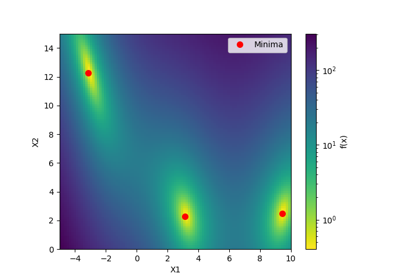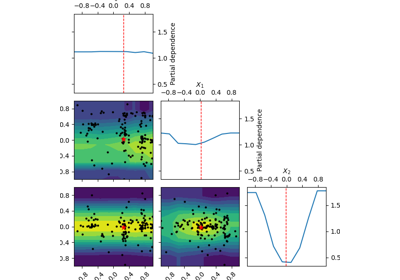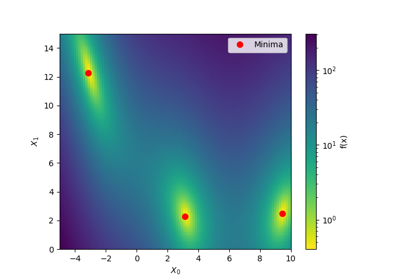# skopt.forest_minimize¶

skopt.forest_minimize(func, dimensions, base_estimator='ET', n_calls=100, n_random_starts=None, n_initial_points=10, acq_func='EI', initial_point_generator='random', x0=None, y0=None, random_state=None, verbose=False, callback=None, n_points=10000, xi=0.01, kappa=1.96, n_jobs=1, model_queue_size=None)[source][source]

Sequential optimisation using decision trees.

A tree based regression model is used to model the expensive to evaluate function func. The model is improved by sequentially evaluating the expensive function at the next best point. Thereby finding the minimum of func with as few evaluations as possible.

The total number of evaluations, n_calls, are performed like the following. If x0 is provided but not y0, then the elements of x0 are first evaluated, followed by n_initial_points evaluations. Finally, n_calls - len(x0) - n_initial_points evaluations are made guided by the surrogate model. If x0 and y0 are both provided then n_initial_points evaluations are first made then n_calls - n_initial_points subsequent evaluations are made guided by the surrogate model.

The first n_initial_points are generated by the initial_point_generator.

Parameters
funccallable

Function to minimize. Should take a single list of parameters and return the objective value.

If you have a search-space where all dimensions have names, then you can use skopt.utils.use_named_args() as a decorator on your objective function, in order to call it directly with the named arguments. See skopt.utils.use_named_args() for an example.

dimensionslist, shape (n_dims,)

List of search space dimensions. Each search dimension can be defined either as

• a (lower_bound, upper_bound) tuple (for Real or Integer dimensions),

• a (lower_bound, upper_bound, prior) tuple (for Real dimensions),

• as a list of categories (for Categorical dimensions), or

• an instance of a Dimension object (Real, Integer or Categorical).

Note

The upper and lower bounds are inclusive for Integer dimensions.

base_estimatorstring or Regressor, default: "ET"

The regressor to use as surrogate model. Can be either

• "RF" for random forest regressor

• "ET" for extra trees regressor

• instance of regressor with support for return_std in its predict method

The predefined models are initialized with good defaults. If you want to adjust the model parameters pass your own instance of a regressor which returns the mean and standard deviation when making predictions.

n_callsint, default: 100

Number of calls to func.

n_random_startsint, default: None

Number of evaluations of func with random points before approximating it with base_estimator.

Deprecated since version 0.8: use n_initial_points instead.

n_initial_pointsint, default: 10

Number of evaluations of func with initialization points before approximating it with base_estimator. Initial point generator can be changed by setting initial_point_generator.

initial_point_generatorstr, InitialPointGenerator instance, default: "random"

Sets a initial points generator. Can be either

• "random" for uniform random numbers,

• "sobol" for a Sobol sequence,

• "halton" for a Halton sequence,

• "hammersly" for a Hammersly sequence,

• "lhs" for a latin hypercube sequence,

• "grid" for a uniform grid sequence

acq_funcstring, default: "LCB"

Function to minimize over the forest posterior. Can be either

• "LCB" for lower confidence bound.

• "EI" for negative expected improvement.

• "PI" for negative probability of improvement.

• "EIps" for negated expected improvement per second to take into account the function compute time. Then, the objective function is assumed to return two values, the first being the objective value and the second being the time taken in seconds.

• "PIps" for negated probability of improvement per second. The return type of the objective function is assumed to be similar to that of "EIps"

x0list, list of lists or None

Initial input points.

• If it is a list of lists, use it as a list of input points.

• If it is a list, use it as a single initial input point.

• If it is None, no initial input points are used.

y0list, scalar or None

Evaluation of initial input points.

• If it is a list, then it corresponds to evaluations of the function at each element of x0 : the i-th element of y0 corresponds to the function evaluated at the i-th element of x0.

• If it is a scalar, then it corresponds to the evaluation of the function at x0.

• If it is None and x0 is provided, then the function is evaluated at each element of x0.

random_stateint, RandomState instance, or None (default)

Set random state to something other than None for reproducible results.

verboseboolean, default: False

Control the verbosity. It is advised to set the verbosity to True for long optimization runs.

callbackcallable, optional

If provided, then callback(res) is called after call to func.

n_pointsint, default: 10000

Number of points to sample when minimizing the acquisition function.

xifloat, default: 0.01

Controls how much improvement one wants over the previous best values. Used when the acquisition is either "EI" or "PI".

kappafloat, default: 1.96

Controls how much of the variance in the predicted values should be taken into account. If set to be very high, then we are favouring exploration over exploitation and vice versa. Used when the acquisition is "LCB".

n_jobsint, default: 1

The number of jobs to run in parallel for fit and predict. If -1, then the number of jobs is set to the number of cores.

model_queue_sizeint or None, default: None

Keeps list of models only as long as the argument given. In the case of None, the list has no capped length.

Returns
resOptimizeResult, scipy object

The optimization result returned as a OptimizeResult object. Important attributes are:

• x [list]: location of the minimum.

• fun [float]: function value at the minimum.

• models: surrogate models used for each iteration.

• x_iters [list of lists]: location of function evaluation for each iteration.

• func_vals [array]: function value for each iteration.

• space [Space]: the optimization space.

• specs [dict]: the call specifications.

For more details related to the OptimizeResult object, refer http://docs.scipy.org/doc/scipy/reference/generated/scipy.optimize.OptimizeResult.html

## Examples using skopt.forest_minimize`¶